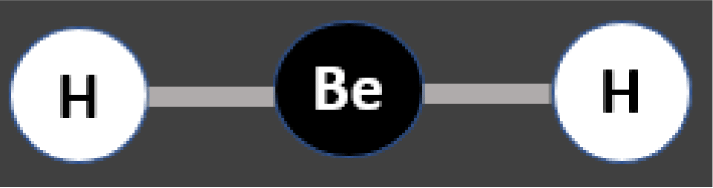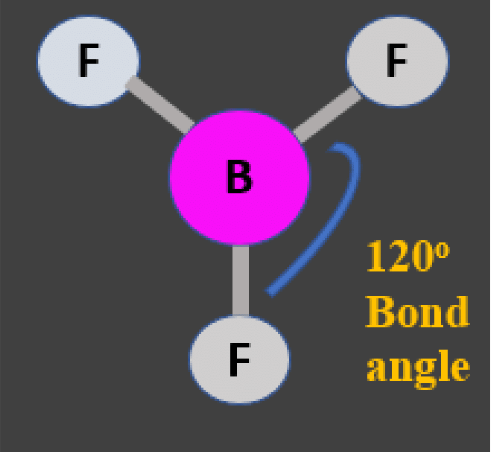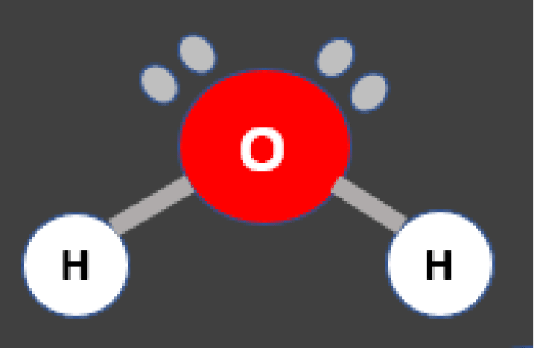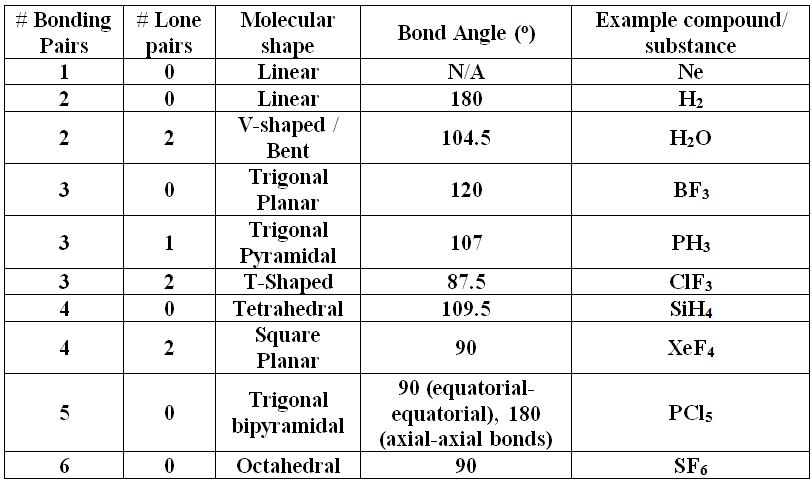# Molecular geometry and VSEPR

### Molecular geometry and VSEPR

#### Lessons

In this lesson, we will learn:
• To understand the ball-and-stick method of describing molecules in 3d space.
• To understand the principles of VSEPR theory.
• To apply VSEPR theory to predict the shapes and bond angles of different molecules.

Notes:
• Molecules and compounds alike are groups of atoms held together by chemical bonds between them. One way to imagine these molecules is a ball and stick model the atoms are spherical balls connected by sticks (the chemical bonds) to each other to form the molecule.

• Molecules are made of atoms connected by covalent bonds.
• A covalent bond is a pair of electrons shared between two atoms. The electrons have a Coulombic attraction to the two nuclei they sit between.
• Electrons repel each other. Electron pairs of any sort will try to place themselves as far away from each other as possible to reduce repulsion.

We can use these to predict the shape of molecules by finding the number of valence (outer shell) electrons the central atom has around it. This can determine both the shape and bond angles around the central atom. This method is known as Valence Shell Electron Pair Repulsion (VSEPR) theory.

• According to VSEPR, the electron domain is what determines the geometry (shape) of a molecule.
There are two contributing factors to the electron domain:
• The number of bonding electron pairs around the central atom. These outer shell electrons will position themselves as far away from each other as possible to reduce electron repulsion. Double and triple covalent bonds count as one electron domain.
• For example, if there were two pairs of bonding electrons around one atom, they would position themselves on opposite sides of the central atom. The angle between the bonds they make would be 180°, which we call the bond angle. The atoms of the molecule would be in a straight line with each other, so the shape is called linear. See below for CO2, a linear shaped compound with two bonding pairs of electrons.

•• If there were three pairs of bonding electrons around the central atom they would divide the same 360° area in three equally spaced angles – 120° each. The three directions the bonds now point and place the atoms they're bonded to form the edges of a flat triangle, so the shape is called trigonal planar. See below for BF3, a trigonal planar molecule with three bonding pairs.

•• The number of pairs of non-bonding (lone pair) electrons around the central atom. Non-bonding pairs cause greater repulsion than bonding pairs because the electrons are localized on one atom rather than being shared by two atoms.
This means that bond angles in molecules with lone pairs are decreased – around 2.5° for each lone pair present.
• Water, H2O, has two bonding pairs and two non-bonding pairs on the central O atom. The normal bond angle of 109.5° for 4 bonding pairs (see below) is reduced by 5° for the angle between the two bonding pairs. The drop from 4 to 2 bonding pairs also means the shape changes theres two less atoms to make a shape with! Both lone pairs repel the bonding pairs down into an angular or bent V shape. See below for the geometry of water, H2O.

•• Molecular shapes and their bond angles can be predicted from finding the number of bonding and lone pairs around a central atom. Many shapes are summarized in this table:

•• Introduction
Working out shapes of molecules
a)
Recap on Lewis (dot and cross) structures.

b)
Using Lewis structures for VSEPR theory.

c)
Factors affecting molecular geometry and bond angle.

d)
Example: 2 bonding pairs

e)
Example: 2 bonding pairs and 2 lone pairs

f)
Example: 3 bonding pairs

g)
Example: 3 bonding pairs and 1 lone pair

h)
Example: 4 bonding pairs

i)
Other examples with 4 bonding pairs

j)
Example: 5 bonding pairs

k)
Example: 6 bonding pairs

l)
Table of molecular geometries and bond angles.

• 1.
Predict the molecular geometry and bond angles of the following molecules:
a)
i) Cl2
ii) AlCl2

b)
i) CH4
ii) NH3

• 2.
Predict the molecular geometry and bond angle of the following molecules
a)
i) PCl5
ii) SF6

b)
H2O

c)
CIF3